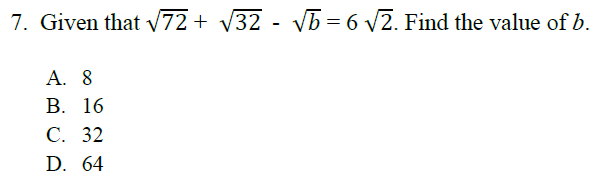2014 Waec Scheme for Mathematics Questions And Answers

# 2014 Waec Scheme for Mathematics Questions And Answers

GENERAL MATHEMATICS/MATHEMATICS (CORE) EXAMINATION SCHEME
There will be two papers, Papers 1 and 2, both of which must be taken.

PAPER 1: will consist of fifty multiple-choice objective questions, drawn from the common areas of the syllabus, to be answered in 1Â½ hours for 50 marks.

PAPER 2: will consist of thirteen essay questions in two sections â€“ Sections A and B, to be answered in 2Â½ hours for 100 marks. Candidates will be required to answer ten questions in all.

Section A - Will consist of five compulsory questions, elementary in nature carrying a total of 40 marks. The questions will be drawn from the common areas of the syllabus.

Section B - will consist of eight questions of greater length and difficulty. The questions shall include a maximum of two which shall be drawn from parts of the syllabuses which may not be peculiar to candidatesâ€™ home countries. Candidates will be expected to answer five questions for 60marks.

SAMPLE QUESTIONS
PAPER 1
(OBJECTIVES)

1. If log10X = 0. 7549 and log10Y = 0.3285, find log10XY.
A. 0.4256
B. 0.2479
C. 1.0826
D. 2.2956

2. Convert 13.025 to base 10.
A. 8.08
B. 8.80
C. 808
D. 880

3. Simplify : 3x+1(2x-1) .
2-1 (6x)
A. 6
B. 3
C. 3x
D. 2x

4. A bonus issue of ordinary shares on the basis of three new shares for every five held has been made by a company. What will be the increase in the number of shares of an investor who originally had 75,000 ordinary shares?
A. 15,000
B. 25,000
C. 45,000
D. 75,000

5. Find the equation of the line which is perpendicular to y = and which passes through the point (1, 3).
A. y = -3x
B. y = 3x
C. y = -3x + 6
D. y = 3x â€“ 6

6. Which of the following is represented by the shaded region of the Venn
diagram below?Related Article

## » Geography WAEC 2014 Questions And Answers

Posted Bykellychi On 08:52 Mon, 10 Mar 2014